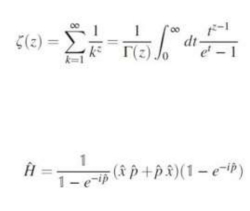# New insight into proving math’s million-dollar problem: the Riemann hypothesis

Lisa Zyga in Phys.org:Researchers have discovered that the solutions to a famous mathematical function called the Riemann zeta function correspond to the solutions of another, different kind of function that may make it easier to solve one of the biggest problems in mathematics: the Riemann hypothesis. If the results can be rigorously verified, then it would finally prove the Riemann hypothesis, which is worth a \$1,000,000 Millennium Prize from the Clay Mathematics Institute.

While the Riemann hypothesis dates back to 1859, for the past 100 years or so mathematicians have been trying to find an operator function like the one discovered here, as it is considered a key step in the proof.

"To our knowledge, this is the first time that an explicit—and perhaps surprisingly relatively simple—operator has been identified whose eigenvalues ['solutions' in matrix terminology] correspond exactly to the nontrivial zeros of the Riemann zeta function," Dorje Brody, a mathematical physicist at Brunel University London and coauthor of the new study, told Phys.org.

What still remains to be proven is the second key step: that all of the eigenvalues are real numbers rather than imaginary ones. If future work can prove this, then it would finally prove the Riemann hypothesis.

More here.